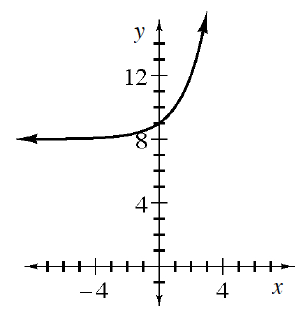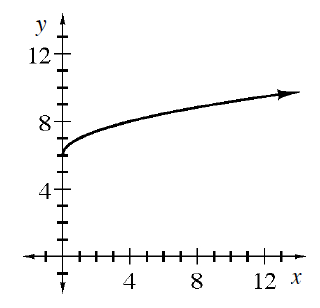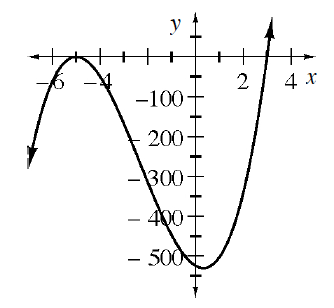### Home > INT3 > Chapter 8 > Lesson 8.1.1 > Problem8-9

8-9.

Which of the following equations and graphs represent polynomial functions? For those that are not polynomials, explain why not. Review the Math Notes box in this lesson for information about polynomials.

1. $f\left(x\right) = 8x^{5} + x^{2} + 6.5x^{4} + 6$

This is a polynomial.

1. $y=\frac{3}{5}x^6+19x^2$

1.This is not a polynomial.

1.1.This is a polynomial.

1. $y = x ^ { 2 } + \frac { 1 } { x ^ { 2 } + 5 }$

1. Write an equation for a new function that is a polynomial and then write an equation for a new function that is not a polynomial.

Look at parts (a) through (f) for examples.